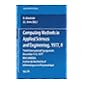Normal view

# Computing methods in applied sciences and engineering, 1977, I / Third International Symposium, December 5-9, 1977 ; [organized by] IRIA LABORIA, Institut de recherche d'informatique et d'automatique ; edited by R. Glowinski and J.L. Lions.

Material type:TextLanguage: English, French Series: Lecture notes in mathematics (Springer-Verlag) ; 704.Publication details: 1979. Description: 1 online resource (vi, 391 pages) : illustrationsContent type: text Media type: computer Carrier type: online resourceISBN: 9783540354116; 3540354115Additional physical formats: Print version:: Computing methods in applied sciences and engineering, 1977, I.DDC classification: 518 LOC classification: QA3 | .L28 no. 704 | QA297Online resources: Click here to access online
Contents:
Sur Certains Aspects Qualitatifs de la Theorie des Equations aux Derivees Partielles -- Les Problems Mal Poses et les Problemes Mathematiques de Traitement Automatique de Resultats d'Experiences -- Iterative methods in numerical solution of differential equations -- Numerical methods for complementarity problems in engineering and applied science -- Optimisation non Differentiable: Methodes de Faisceaux -- Variable metric methods for constrained optimization -- Constant strain finite elements -- Computation of eddy currents on a surface in ?3 by finite element methods -- The club MODULEF -- Finite element analysis for stress intensity factors -- The sommerfeld (radiation) condition on infinite domains and its modelling in numerical procedures -- On the finite element approximation for evolution equations of parabolic type -- Special applications of Hamiltons's principle to structural dynamics -- Constructive methods for bifurcation and nonlinear eigenvalue problems -- Finite element approximations to bifurcation problems of turning point type -- On numerical deformation of singularities in nonlinear elasticity -- Numerical methods for free surface problems by means of penalty -- A family of model problems in plasticity -- The computational aspects of the homogenization problem -- Asymptotics for branching transport processes -- Numerical experiments of the homogenization method -- A homogenized multigroup diffusion theory for the neutron transport equation -- Estimation de Coefficients Homogenises -- Some applications of computational mathematics to medical problems.
Action note: digitized 2010 committed to preserve
Tags from this library: No tags from this library for this title.
Star ratingsAverage rating: 0.0 (0 votes)
Holdings
Item type Current library Collection Call number Status Date due Barcode Item holdseBook e-Library

Electronic Book@IST

EBook Available
Total holds: 0

Includes bibliographical references.

Use copy Restrictions unspecified star MiAaHDL

Electronic reproduction. [Place of publication not identified] : HathiTrust Digital Library, 2010. MiAaHDL

Master and use copy. Digital master created according to Benchmark for Faithful Digital Reproductions of Monographs and Serials, Version 1. Digital Library Federation, December 2002. MiAaHDL

digitized 2010 HathiTrust Digital Library committed to preserve pda MiAaHDL

Print version record.

Sur Certains Aspects Qualitatifs de la Theorie des Equations aux Derivees Partielles -- Les Problems Mal Poses et les Problemes Mathematiques de Traitement Automatique de Resultats d'Experiences -- Iterative methods in numerical solution of differential equations -- Numerical methods for complementarity problems in engineering and applied science -- Optimisation non Differentiable: Methodes de Faisceaux -- Variable metric methods for constrained optimization -- Constant strain finite elements -- Computation of eddy currents on a surface in ?3 by finite element methods -- The club MODULEF -- Finite element analysis for stress intensity factors -- The sommerfeld (radiation) condition on infinite domains and its modelling in numerical procedures -- On the finite element approximation for evolution equations of parabolic type -- Special applications of Hamiltons's principle to structural dynamics -- Constructive methods for bifurcation and nonlinear eigenvalue problems -- Finite element approximations to bifurcation problems of turning point type -- On numerical deformation of singularities in nonlinear elasticity -- Numerical methods for free surface problems by means of penalty -- A family of model problems in plasticity -- The computational aspects of the homogenization problem -- Asymptotics for branching transport processes -- Numerical experiments of the homogenization method -- A homogenized multigroup diffusion theory for the neutron transport equation -- Estimation de Coefficients Homogenises -- Some applications of computational mathematics to medical problems.

## Other editions of this workComputing methods in applied sciences : ©1976Computing methods in applied sciences and engineering, 1977, II / ©1979
Share